﻿
Common Core Math for Kindergarten: Counting and Cardinality | Student HandoutsKindergarten Math: Counting and Cardinality
www.studenthandouts.com > Kindergarten > Kindergarten Math > Kindergarten Counting/Cardinality

CCSS.MATH.CONTENT.K.CC.A.1 - Count to 100 by ones and tens.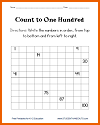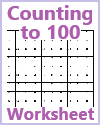Count and Write Numerals to 100 Counting to 100 Worksheet

CCSS.MATH.CONTENT.K.CC.A.2 - Count forward beginning from a given number within the known sequence (instead of having to begin at 1).

CCSS.MATH.CONTENT.K.CC.A.3 - Write numbers from 0 to 20. Represent a number of objects with a written numeral 0-20 (with 0 representing a count of no objects).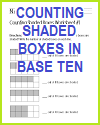Numbers Memory-Style Card Game Counting Shaded Boxes Worksheets Numbers Workbook
CCSS.MATH.CONTENT.K.CC.B.4 - Understand the relationship between numbers and quantities; connect counting to cardinality.

CCSS.MATH.CONTENT.K.CC.B.4.A - When counting objects, say the number names in the standard order, pairing each object with one and only one number name and each number name with one and only one object.

CCSS.MATH.CONTENT.K.CC.B.4.B - Understand that the last number name said tells the number of objects counted. The number of objects is the same regardless of their arrangement or the order in which they were counted.

CCSS.MATH.CONTENT.K.CC.B.4.C - Understand that each successive number name refers to a quantity that is one larger.

CCSS.MATH.CONTENT.K.CC.B.5 - Count to answer "how many?" questions about as many as 20 things arranged in a line, a rectangular array, or a circle, or as many as 10 things in a scattered configuration; given a number from 1-20, count out that many objects.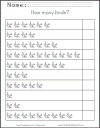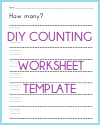Counting Leprechauns Worksheet for St. Patrick's Day How many dreidels? Math Counting Worksheet for Hanukkah How many birds? How many? DIY Counting Worksheet Template How many cylinders?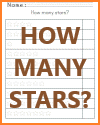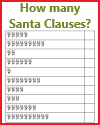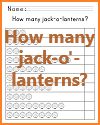How many apples? How many ninjas? How many stars? How many Santa Clauses? How many jack-o'-lanterns? Counting Worksheet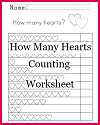"How many hearts?" Counting Worksheet
CCSS.MATH.CONTENT.K.CC.C.6 - Identify whether the number of objects in one group is greater than, less than, or equal to the number of objects in another group, e.g., by using matching and counting strategies.

CCSS.MATH.CONTENT.K.CC.C.7 - Compare two numbers between 1 and 10 presented as written numerals.

www.studenthandouts.com > Kindergarten > Kindergarten Math > Kindergarten Counting/Cardinality